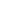# A man travels at a speed of 6 km/hr for five hours and at a speed of 4 km/hr for 10 hours. His average speed is

a) 5
b) 4 2/3
c) 4 1/2
d) 4 1/3
LDC 2010-Malappuram

 2/3. Solution Average speed = Total distance/Total time = (6x5) + (4x10) = 70km Total Time = 5+10 = 15 Average speed = 70/15 = 4 2/3
Share :
+
Next
« Prev
Prev
Next »